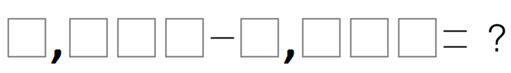# Place Value, Rounding, Addition, and Subtraction

## Objective

Fluently subtract multi-digit whole numbers using the standard algorithm involving multiple decompositions. Solve one-step word problems involving subtraction.

## Common Core Standards

### Core Standards

?

• 4.NBT.B.4 — Fluently add and subtract multi-digit whole numbers using the standard algorithm.

?

• 3.NBT.A.2

## Criteria for Success

?

1. Subtract multi-digit numbers with multiple decompositions.
2. Solve one-step word problems involving subtraction with multiple decompositions, using a letter to represent the unknown (MP.4).

## Tips for Teachers

?

Students have solved one-step word problems involving subtraction in previous grade levels (1.OA.1, 2.OA.1, 3.OA.8), so the intention here is to have students solve contextual problems that involve computations expected of this grade level (4.NBT.4) as well as prepare students to solve multi-step word problems involving addition and subtraction later in the unit, and eventually multiplication (Unit 3) and division (Unit 4) (4.OA.3).

#### Remote Learning Guidance

If you need to adapt or shorten this lesson for remote learning, we suggest prioritizing Anchor Task 2 (benefits from worked example). Find more guidance on adapting our math curriculum for remote learning here.

#### Fishtank Plus

• Problem Set
• Student Handout Editor
• Vocabulary Package

?

### Problem 1

Place any digit, 1 through 9, in the boxes below to create the smallest possible difference. Each digit can only be used once.#### References

Open Middle Subtraction to Get the Smallest Difference

Subtraction to Get the Smallest Difference by is made available on Open Middle under the CC BY-NC-SA 4.0 license. Accessed Sept. 13, 2017, 8:46 a.m..

Modified by The Match Foundation, Inc.

### Problem 2

Estimate. Then solve.

1.    253,421 – $\square$  = 75,832

1.      1,000,000

-   978,564

#### References

EngageNY Mathematics Grade 4 Mathematics > Module 1 > Topic E > Lesson 15Concept Development

Grade 4 Mathematics > Module 1 > Topic E > Lesson 15 of the New York State Common Core Mathematics Curriculum from EngageNY and Great Minds. © 2015 Great Minds. Licensed by EngageNY of the New York State Education Department under the CC BY-NC-SA 3.0 US license. Accessed Dec. 2, 2016, 5:15 p.m..

Modified by The Match Foundation, Inc.

### Problem 3

Last year, there were 620,073 people in attendance at a local parade. There were 163,278 more at the parade last year than there were this year. How many people were in attendance this year?

#### References

EngageNY Mathematics Grade 4 Mathematics > Module 1 > Topic E > Lesson 15Concept Development

Grade 4 Mathematics > Module 1 > Topic E > Lesson 15 of the New York State Common Core Mathematics Curriculum from EngageNY and Great Minds. © 2015 Great Minds. Licensed by EngageNY of the New York State Education Department under the CC BY-NC-SA 3.0 US license. Accessed Dec. 2, 2016, 5:15 p.m..

Modified by The Match Foundation, Inc.

## Problem Set & Homework

#### Discussion of Problem Set

• Look at #1(d). If you had added 13 to both numbers, would it have resulted in the same difference? What is the advantage of adding 13 to both numbers?
• Look at #1(e) and 1(f). Did anyone notice a pattern that could be used to solve these problems?
• Did anyone count up to solve #1(g)? Why did you decide to do that? What is the advantage of that strategy?
• What was different about #1(i) than the previous problems?
• What mistake did the student make in #4? How would you correct it? What is the correct difference?
• Look at #5 and #6. How are these problems similar? How are they different?
• How do you know when you are ready to subtract across the problem?
• Is there a number that you can subtract from 1,000,000 without decomposing across to the ones (other than 1,000,000)? 100,000? 10,000?
• How can decomposing multiple times be challenging?
• How does the tape diagram help you determine which operation to use to find the answer?

?

Solve. Show or explain your work.

1.    $\square$ = 32,010 – 2,546
2.    956,204 = 780,169 + _______________
3.    A construction company was building a stone wall on Main Street. 100,000 stones were delivered to the site. On Monday, they used 15,631 stones. How many stones remain for the rest of the week? Show or explain your work.

#### References

EngageNY Mathematics Grade 4 Mathematics > Module 1 > Topic E > Lesson 15Exit Ticket

Grade 4 Mathematics > Module 1 > Topic E > Lesson 15 of the New York State Common Core Mathematics Curriculum from EngageNY and Great Minds. © 2015 Great Minds. Licensed by EngageNY of the New York State Education Department under the CC BY-NC-SA 3.0 US license. Accessed Dec. 2, 2016, 5:15 p.m..

Modified by The Match Foundation, Inc.

### Mastery Response

?